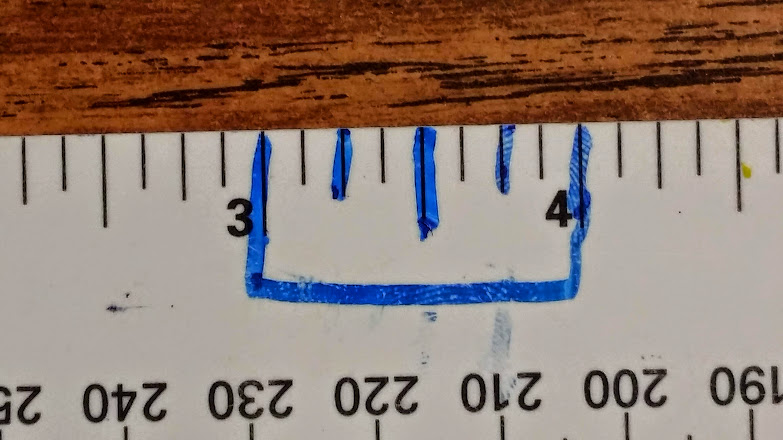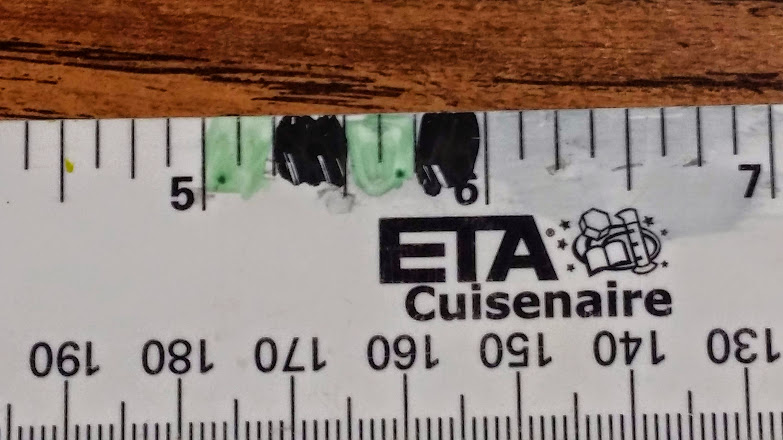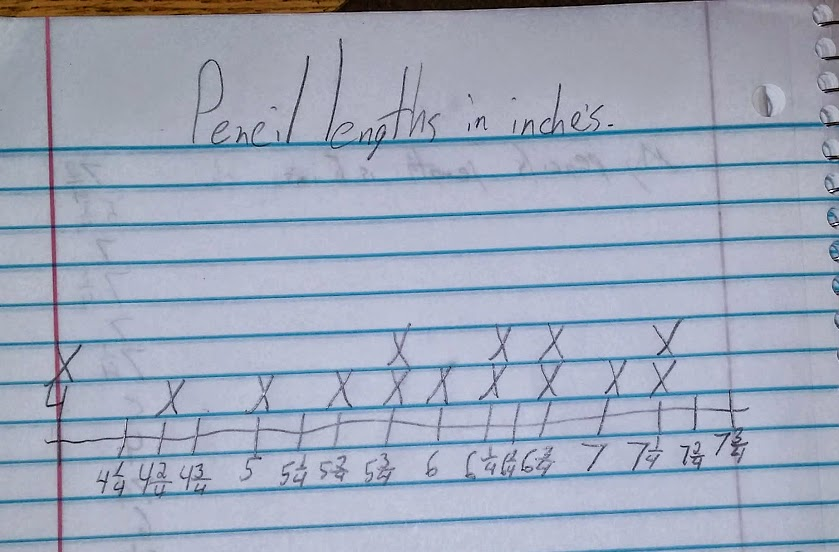# Pencils, Bugs and Line Plots - Day 1

14 teachers like this lesson
Print Lesson

## Objective

SWBAT measure objects to the nearest 1/4, 1/2, or 1/8 inch and create accurate line plots to display the data.

#### Big Idea

In this lessons, students create line plots after measuring objects to the nearest 1/8 of an inch.

## Warm Up

5 minutes

For this warm up, I display this problem for students to solve on their whiteboards.

Which is greater?

8/12  or 6/8

Prove you are right using numbers!!

This is a review problem for students which addresses previously learned fraction concepts.  I wanted students to use number, or common numerators and/or common denominators for this problem because standard 4.NF.2 states that students will compare two fractions by creating common denominators or numerators.  Many of my students have become experts at using models to compare fractions, and are less reluctant to use numbers.  I wanted to stress the importance of using a number strategy.

## Concept Development

45 minutes

I begin this lesson by asking students what they remember about line plots from a previous lesson. Students respond with saying things like: line plots show how often something occurs, or how many times something happened.  I remind students that line plots do show frequency.

Students work on 4.MD.4 in this lesson.  I begin by asking students how to measure an object to the nearest quarter of an inch.  Many of my students have had experiences measuring objects to the nearest quarter and half inch, though some have not.  It is important that I ensure all students know how to measure to the nearest quarter inch.

Next, I spend some time showing the ruler that students will use and we practice measuring on object together. One way I show students fourths is by drawing the four equal parts on the ruler. I tell students that I don't want them to draw on their ruler, but we practice using mine.  (In the future, using a paper ruler as a differentiation tool, in which students could draw on would be something I would definitely have available)

You can see in the two photos one way I mark the ruler for students to see fourths or four equal parts.Next, I direct students to use their ruler and measure the length of one of their pencils to the nearest quarter of an inch. I have  students use the pencil that they have been writing with so there will be some variability. Students measure their pencil to the nearest quarter inch.  Then, I direct students to check the measurement with their learning partner.  Once partners agree on both pencil lengths, I ask each student to write their measurements on the white board.

I tell students that their task is to create a line plot with the pencil length data. I lead a brief discussion about some strategies that could be used in order to create a line plot, (ie., arrange the data  from least to greatest, copy all data into your notebook, etc)

Next I give students time to create their line plots. After most students are finished, I lead a discussion about how the line plots were created, what the data means, and how the plot can be interpreted.  Students take turns telling the class something the data shows. (one person had a pencil length of ____, two pencils laid end to end might equal _____inch,  more people had lengths that were reported as fractions rather than whole inches, etc)

If students have trouble coming up with questions, I pose questions like: If we were to select two pencils and place them end-to-end, what are some of the lengths we could obtain? What is the shortest length? What is the longest? What length came up the most? What is the difference between the longest and shortest pencil? Why do you think there are so many different lengths?

You can see in this video below a student  measuring a pencil and hear the questions and prompts I use with this student.

You can see in this photo a student's  finished line plot.  Students noticed that the uneven spacing between the numbers on this specific line plot was some what deceiving. This led students to the conclusion about accuracy being important when making line plots.What is written as the remainder of this lesson, we DID NOT do.  I left it here so you can see how ambitious I was in thinking my students would do two lines plots in a 45 minute time frame.  This will become part of the next day's lesson.

For the next part of the lesson, I pair the students and ask them to select a bug from the bug sheet. (bugs)

Next, I direct students and tell them that each of the partners measure the bug to the nearest eighth of an inch. Once they have agreed on the length, they should put the length on the board. Partners should then work together to create a line plot of the whole class’s data. Partners could then compare their plots with another pair of students. Each pair would then create questions for the other students in the class to answer based on the line plot. Spend time sharing and answering the questions.